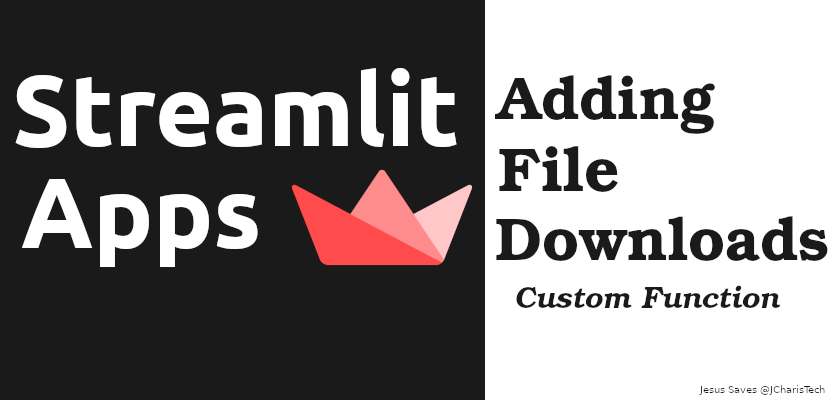By the end of this tutorial you will learn how to

• Based64 Encode & Decode Data
• etc

Currently (circa 2021) there is no widget natively in streamlit to download files however streamlit is flexible. It offers a lot of cool features that can enable as to hack this feature out.

We will be using base64 library and streamlit markdown feature to enable us add file downloads to our app.

The secret behind file downloads in our app is using base64 to encode and decode our data in order to send it over the wire via an anchor tag (a href). Credit to the amazing developers on the streamlit forums for this function.

Let us see how to achieve that. We will be using two functions and then we will create a reusable class object to do the same.

``````def text_downloader(raw_text):
b64 = base64.b64encode(raw_text.encode()).decode()
new_filename = "new_text_file_{}_.txt".format(timestr)
st.markdown(href,unsafe_allow_html=True)``````

Let us break down the code

The next line allows us to have a file name with a timestamp for convenience. We will then send the encoded variable via href to make it downloadable as a link.

Using streamlit.markdown() we will render the anchor tag as a link. Setting unsafe_allow_html =True enables the a href tag to be rendered correctly.

This same function can be applied for CSV file with the help of pandas.to_csv() , we can convert the data into a format we can base64 encode. Below is the code for that

``````def csv_downloader(data):
csvfile = data.to_csv()
b64 = base64.b64encode(csvfile.encode()).decode()
new_filename = "new_text_file_{}_.csv".format(timestr)
st.markdown(href,unsafe_allow_html=True)``````

Moreover you can convert these functions into a class object as below

``````# Class

"""
def __init__(self, data,filename='myfile',file_ext='txt'):
self.data = data
self.filename = filename
self.file_ext = file_ext

b64 = base64.b64encode(self.data.encode()).decode()
new_filename = "{}_{}_.{}".format(self.filename,timestr,self.file_ext)
st.markdown(href,unsafe_allow_html=True)``````

We can check the whole code for the app below

``````import streamlit as st
import streamlit.components as stc

# Utils
import base64
import time
timestr = time.strftime("%Y%m%d-%H%M%S")
import pandas as pd

# Fxn
b64 = base64.b64encode(raw_text.encode()).decode()
new_filename = "new_text_file_{}_.txt".format(timestr)
st.markdown(href,unsafe_allow_html=True)

csvfile = data.to_csv()
b64 = base64.b64encode(csvfile.encode()).decode()
new_filename = "new_text_file_{}_.csv".format(timestr)
st.markdown(href,unsafe_allow_html=True)

# Class

"""
def __init__(self, data,filename='myfile',file_ext='txt'):
self.data = data
self.filename = filename
self.file_ext = file_ext

b64 = base64.b64encode(self.data.encode()).decode()
new_filename = "{}_{}_.{}".format(self.filename,timestr,self.file_ext)
st.markdown(href,unsafe_allow_html=True)

def main():

if choice == "Home":
if st.button("Save"):
st.write(my_text)

elif choice == "CSV":
st.dataframe(df)

else: